Number T of arithmetic operations that guarantees the required accuracy of solution and memory volume M required for the implementation of the algorithm are the main efficiency criteria for any numerical method. To solve differential equations (with boundary condition and radiation condition) and integral equations (taking into account discretization method) we estimate input data T and M. Afterwards on the base of estimations obtained we analyze the efficiency of using differential or integral equations for the abovementioned problems.

ご不明な点がございましたら、事務局: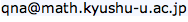までお問い合わせ下さい。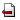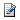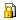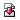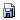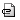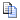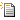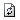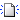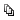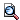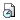Last-modified: 2010-11-12 (金) 14:09:55 (3201d)